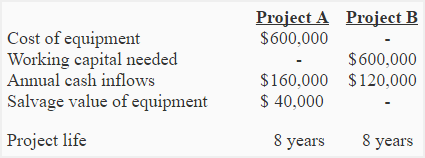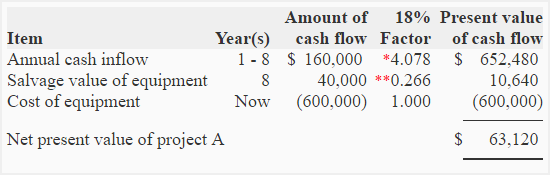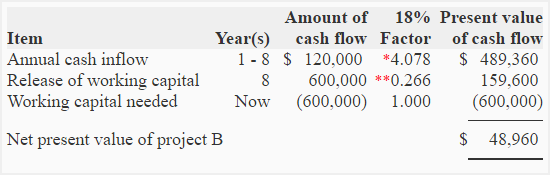# Exercise-16 (Net present value analysis of two alternatives)

The Sunshine company is considering two projects, project A and project B. Project A requires the purchase of an equipment but no working capital investment whereas project B requires a working capital investment but no equipment. The relevant information for net present value analysis is given below:The working capital required for project B will be released at the end of project life. Sunshine company uses an 18% discount rate.

Required: Are the two projects comparable using net present value (NPV)? If yes, Select the best investment using net present value (NPV) method. (Ignore income tax).

## Solution:

Yes, the two projects are comparable because both the projects require equal amount of initial investment.

NPV of project A:*Value from “present value of an annuity of \$1 in arrears table“.
**Value from “present value of \$1 table”.

NPV of project B:*Value from “present value of an annuity of \$1 in arrears table“.
**Value from “present value of \$1 table“.

Conclusion:

According to NPV method, project A looks more desirable because its net present value is more than project B.

A D V E R T I S E M E N T
8 Comments on Exercise-16 (Net present value analysis of two alternatives)
1.Eugenator

Thanx guys keep up the good work.You have always been a way out

2.Densetsu No

Hi there,
I tried to replicate the NPV and found a result of 63.052 for the first one and 48.931 for the second one.
I’m pretty worried that I have calculated the NPV in the wrong way for a long time.
Could you please tell me which formula did you use?

Thanks a lot,
Densetsu

3.Accounting For Management

It may be a rounding difference. Did you use a software or calculator to find NPV of two projects?

1.annie

how if it said that you can borrow fund from the bank at 3% and there are estimated cash inflow and cash outflows.

4.An Hong Nhung

I also had the same result as Densetsu.

I used excel to calculate, just normal simple formulas.

5.ASIM

Sir,

I have list some question related to exercise 16 requested too kindly ans me.

1) Depreciation is not calculated as we can caculate through straight line method ? Why? when we need to calculate depreciation?
2) Working capital will always release in every question or it also depend on question to tell us to release?
3)Solvage value in project B is not calculated why?

6.Accounting For Management

@Asim
(1). Because no tax rate is given i.e., question ignores the income tax.
7.